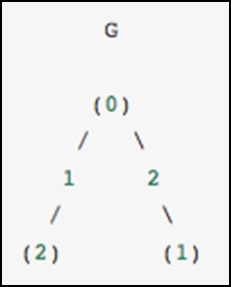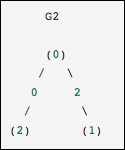# SciPy - CSGraph

CSGraph stands for Compressed Sparse Graph, which focuses on Fast graph algorithms based on sparse matrix representations.

## Graph Representations

To begin with, let us understand what a sparse graph is and how it helps in graph representations.

### What exactly is a sparse graph?

A graph is just a collection of nodes, which have links between them. Graphs can represent nearly anything − social network connections, where each node is a person and is connected to acquaintances; images, where each node is a pixel and is connected to neighboring pixels; points in a high-dimensional distribution, where each node is connected to its nearest neighbors; and practically anything else you can imagine.

One very efficient way to represent graph data is in a sparse matrix: let us call it G. The matrix G is of size N x N, and G[i, j] gives the value of the connection between node ‘i' and node ‘j’. A sparse graph contains mostly zeros − that is, most nodes have only a few connections. This property turns out to be true in most cases of interest.

The creation of the sparse graph submodule was motivated by several algorithms used in scikit-learn that included the following −

• Isomap − A manifold learning algorithm, which requires finding the shortest paths in a graph.

• Hierarchical clustering − A clustering algorithm based on a minimum spanning tree.

• Spectral Decomposition − A projection algorithm based on sparse graph laplacians.

As a concrete example, imagine that we would like to represent the following undirected graph −This graph has three nodes, where node 0 and 1 are connected by an edge of weight 2, and nodes 0 and 2 are connected by an edge of weight 1. We can construct the dense, masked and sparse representations as shown in the following example, keeping in mind that an undirected graph is represented by a symmetric matrix.

```G_dense = np.array([ [0, 2, 1],
[2, 0, 0],
[1, 0, 0] ])

from scipy.sparse import csr_matrix

G_sparse = csr_matrix(G_dense)
print G_sparse.data
```

The above program will generate the following output.

```array([2, 1, 2, 1])
```This is identical to the previous graph, except nodes 0 and 2 are connected by an edge of zero weight. In this case, the dense representation above leads to ambiguities − how can non-edges be represented, if zero is a meaningful value. In this case, either a masked or a sparse representation must be used to eliminate the ambiguity.

Let us consider the following example.

```from scipy.sparse.csgraph import csgraph_from_dense
G2_data = np.array
([
[np.inf, 2, 0 ],
[2, np.inf, np.inf],
[0, np.inf, np.inf]
])
G2_sparse = csgraph_from_dense(G2_data, null_value=np.inf)
print G2_sparse.data
```

The above program will generate the following output.

```array([ 2., 0., 2., 0.])
```

### Word ladders using sparse graphs

Word ladders is a game invented by Lewis Carroll, in which words are linked by changing a single letter at each step. For example −

APE → APT → AIT → BIT → BIG → BAG → MAG → MAN

Here, we have gone from "APE" to "MAN" in seven steps, changing one letter each time. The question is - Can we find a shorter path between these words using the same rule? This problem is naturally expressed as a sparse graph problem. The nodes will correspond to individual words, and we will create connections between words that differ by at the most – one letter.

## Obtaining a List of Words

First, of course, we must obtain a list of valid words. I am running Mac, and Mac has a word dictionary at the location given in the following code block. If you are on a different architecture, you may have to search a bit to find your system dictionary.

```wordlist = open('/usr/share/dict/words').read().split()
print len(wordlist)
```

The above program will generate the following output.

```235886
```

We now want to look at words of length 3, so let us select just those words of the correct length. We will also eliminate words, which start with upper case (proper nouns) or contain non-alpha-numeric characters such as apostrophes and hyphens. Finally, we will make sure everything is in lower case for a comparison later on.

```word_list = [word for word in word_list if len(word) == 3]
word_list = [word for word in word_list if word.islower()]
word_list = [word for word in word_list if word.isalpha()]
word_list = map(str.lower, word_list)
print len(word_list)
```

The above program will generate the following output.

```1135
```

Now, we have a list of 1135 valid three-letter words (the exact number may change depending on the particular list used). Each of these words will become a node in our graph, and we will create edges connecting the nodes associated with each pair of words, which differs by only one letter.

```import numpy as np
word_list = np.asarray(word_list)

word_list.dtype
word_list.sort()

word_bytes = np.ndarray((word_list.size, word_list.itemsize),
dtype = 'int8',
buffer = word_list.data)
print word_bytes.shape
```

The above program will generate the following output.

```(1135, 3)
```

We will use the Hamming distance between each point to determine, which pairs of words are connected. The Hamming distance measures the fraction of entries between two vectors, which differ: any two words with a hamming distance equal to 1/N1/N, where NN is the number of letters, which are connected in the word ladder.

```from scipy.spatial.distance import pdist, squareform
from scipy.sparse import csr_matrix
hamming_dist = pdist(word_bytes, metric = 'hamming')
graph = csr_matrix(squareform(hamming_dist < 1.5 / word_list.itemsize))
```

When comparing the distances, we do not use equality because this can be unstable for floating point values. The inequality produces the desired result as long as no two entries of the word list are identical. Now, that our graph is set up, we will use the shortest path search to find the path between any two words in the graph.

```i1 = word_list.searchsorted('ape')
i2 = word_list.searchsorted('man')
print word_list[i1],word_list[i2]
```

The above program will generate the following output.

```ape, man
```

We need to check that these match, because if the words are not in the list there will be an error in the output. Now, all we need is to find the shortest path between these two indices in the graph. We will use dijkstra’s algorithm, because it allows us to find the path for just one node.

```from scipy.sparse.csgraph import dijkstra
distances, predecessors = dijkstra(graph, indices = i1, return_predecessors = True)
print distances[i2]
```

The above program will generate the following output.

```5.0
```

Thus, we see that the shortest path between ‘ape’ and ‘man’ contains only five steps. We can use the predecessors returned by the algorithm to reconstruct this path.

```path = []
i = i2

while i != i1:
path.append(word_list[i])
i = predecessors[i]

path.append(word_list[i1])
print path[::-1]i2]
```

The above program will generate the following output.

```['ape', 'ope', 'opt', 'oat', 'mat', 'man']
```